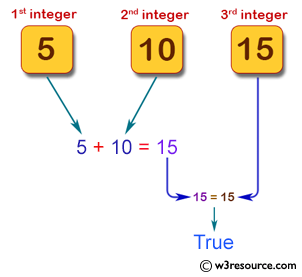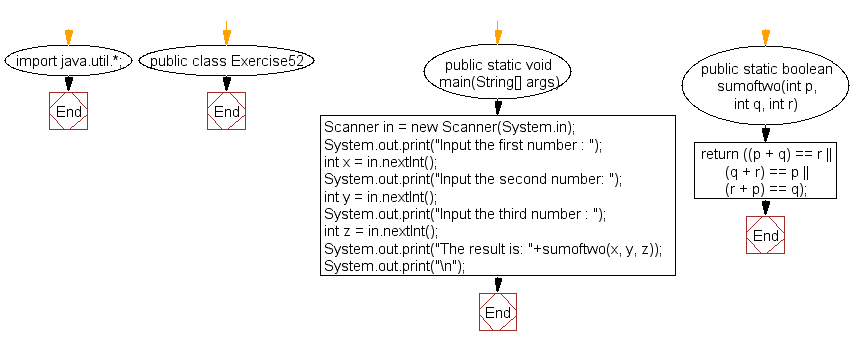﻿ Java: If the sum of two integers is equal to a third# Java Exercises: Calculate the sum of two integers and return true if the sum is equal to a third integer

## Java Basic: Exercise-52 with Solution

Write a Java program to calculate the sum of two integers and return true if the sum is equal to a third integer.

Sample Solution:

Java Code:

``````import java.util.*;
public class Exercise52 {
public static void main(String[] args)
{
Scanner in = new Scanner(System.in);
System.out.print("Input the first number : ");
int x = in.nextInt();
System.out.print("Input the second number: ");
int y = in.nextInt();
System.out.print("Input the third number : ");
int z = in.nextInt();
System.out.print("The result is: "+sumoftwo(x, y, z));
System.out.print("\n");
}

public static boolean sumoftwo(int p, int q, int r)
{
return ((p + q) == r || (q + r) == p || (r + p) == q);
}
}
```
```

Sample Output:

```Input the first number : 5
Input the second number: 10
Input the third number : 15
The result is: true
```

Pictorial Presentation:Flowchart:Java Code Editor:

What is the difficulty level of this exercise?

Test your Programming skills with w3resource's quiz.

﻿

## Java: Tips of the Day

getEnumMap

Converts to enum to Map where key is the name and value is Enum itself.

```public static <E extends Enum<E>> Map<String, E> getEnumMap(final Class<E> enumClass) {
return Arrays.stream(enumClass.getEnumConstants())
.collect(Collectors.toMap(Enum::name, Function.identity()));
}
```

Ref: https://bit.ly/3xXcFZt